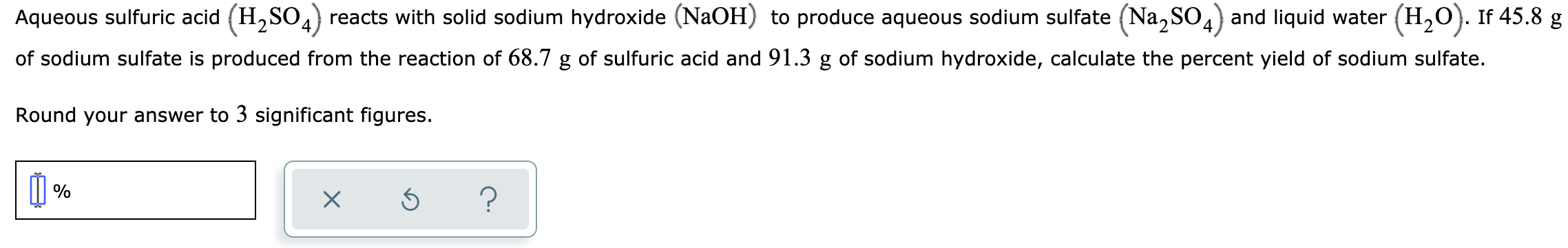# Aqueous sulfuric acid (H,SO,) reacts with solid sodium hydroxide (NaOH) to produce aqueous sodium sulfate (Na, SO,) and liquid water (H,0). If 45.8 g of sodium sulfate is produced from the reaction of 68.7 g of sulfuric acid and 91.3 g of sodium hydroxide, calculate the percent yield of sodium sulfate. Round your answer to 3 significant figures.

Questionhelp_outlineImage TranscriptioncloseAqueous sulfuric acid (H,SO,) reacts with solid sodium hydroxide (NaOH) to produce aqueous sodium sulfate (Na, SO,) and liquid water (H,0). If 45.8 g of sodium sulfate is produced from the reaction of 68.7 g of sulfuric acid and 91.3 g of sodium hydroxide, calculate the percent yield of sodium sulfate. Round your answer to 3 significant figures. fullscreen